# Important Questions Class 10 Maths Chapter 9 Applications of Trigonometry

Important questions for Class 10 Maths Chapter 9 Some Applications of Trigonometry are provided here for the board exams preparation. The questions are based on the new pattern of CBSE and are as per the revised syllabus. Students who are preparing CBSE 2022-2023 Maths exam are advised to practice these important questions of Some Applications of Trigonometry For Class 10. Solving these questions will help students to score high marks in the questions asked from this chapter.

Trigonometry has more applications in our daily existence, and hence, the chapter is crucial for the board exam and valuable in many other fields. Most of the questions from this chapter are also asked in the competitive exams such as JEE etc.

Below, we have provided the questions of Chapter 9 Applications of Trigonometry with the solutions. Students can also find additional questions without solutions for their practice.

## Important Questions & Answers For Class 10 Maths Chapter 9 – Some Applications of Trigonometry

Q.1: The shadow of a tower standing on level ground is found to be 40 m longer when the Sun’s altitude is 30° than when it is 60°. Find the height of the tower.

Solution:

Let AB be the tower and BC be the length of its shadow when the sun’s altitude (angle of elevation from the top of the tower to the tip of the shadow) is 60° and DB be the length of the shadow when the angle of elevation is 30°.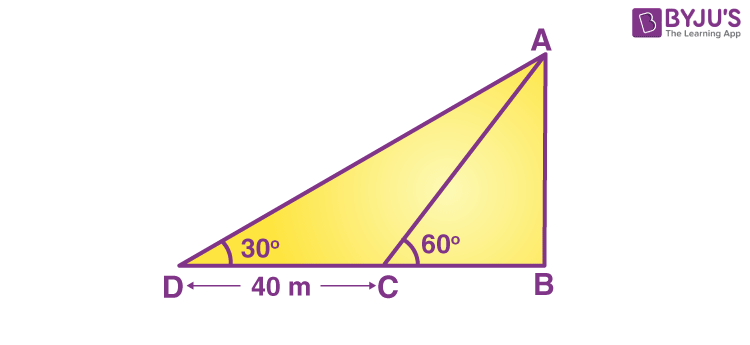Let us assume, AB = h m, BC = x m

DB = (40 +x) m

In the right triangle ABC,

tan 60° = AB/BC

√3 = h/x

h = √3 x……….(i)

In the right triangle ABD,

tan 30° = AB/BD

1/√3 =h/(x + 40) ……..(ii)

From (i) and (ii),

x(√3 )(√3 ) = x + 40

3x = x + 40

2x = 40

x = 20

Substituting x = 20 in (i),

h = 20√3

Hence, the height of the tower is 20√3 m.

Q. 2: A tree breaks due to a storm and the broken part bends so that the top of the tree touches the ground making an angle of 30° with it. The distance between the foot of the tree to the point where the top touches the ground is 8 m. Find the height of the tree.

Solution:

Using given instructions, draw a figure. Let AC be the broken part of the tree. Angel C = 30 degrees.

BC = 8 m

To Find: Height of the tree, which is AB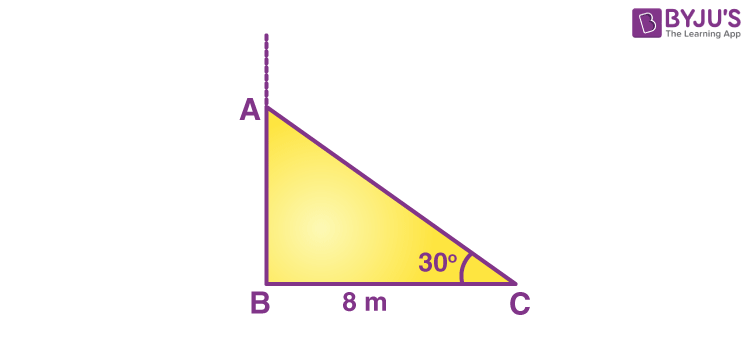From figure: Total height of the tree is the sum of AB and AC i.e. AB+AC

In right ΔABC,

Using Cosine and tangent angles,

cos 30° = BC/AC

We know that, cos 30° = √3/2

√3/2 = 8/AC

AC = 16/√3 …(1)

Also,

tan 30° = AB/BC

1/√3 = AB/8

AB = 8/√3 ….(2)

From (1) and (2),

Total height of the tree = AB + AC = 16/√3 + 8/√3 = 24/√3 = 8√3 m.

Q. 3: Two poles of equal heights are standing opposite each other on either side of the road, which is 80 m wide. From a point between them on the road, the angles of elevation of the top of the poles are 60° and 30°, respectively. Find the height of the poles and the distances of the point from the poles.

Solution:

Let AB and CD be the poles of equal height.

O is the point between them from where the height of elevation is taken. BD is the distance between the poles.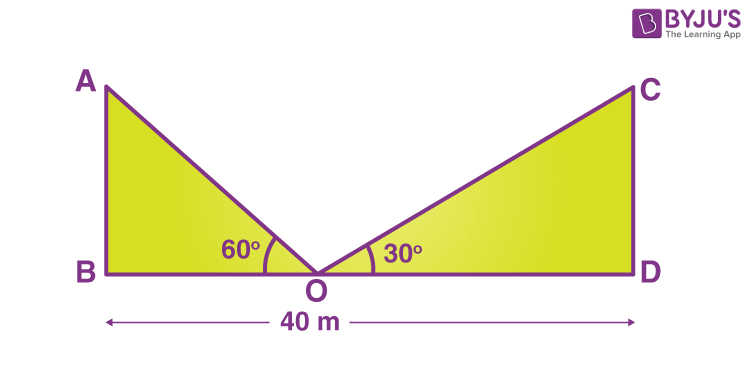As per the above figure, AB = CD,

OB + OD = 80 m

Now,

In right ΔCDO,

tan 30° = CD/OD

1/√3 = CD/OD

CD = OD/√3 … (1)

In right ΔABO,

tan 60° = AB/OB

√3 = AB/(80-OD)

AB = √3(80-OD)

AB = CD (Given)

√3(80-OD) = OD/√3 (Using equation (1))

3(80-OD) = OD

240 – 3 OD = OD

4 OD = 240

OD = 60

Substituting the value of OD in equation (1)

CD = OD/√3

CD = 60/√3

CD = 20√3 m

Also,

OB + OD = 80 m

⇒ OB = (80-60) m = 20 m

Therefore, the height of the poles are 20√3 m and the distance from the point of elevation are 20 m and 60 m respectively.

Q. 4: An observer 1.5 metres tall is 20.5 metres away from a tower 22 metres high. Determine the angle of elevation of the top of the tower from the eye of the observer.

Solution:

Let AB be the height of the observer and PR be the height of the tower.

Also, PB is the distance between the foot of the tower and the observer.

Consider θ as the angle of elevation of the top of the tower from the eye of the observer.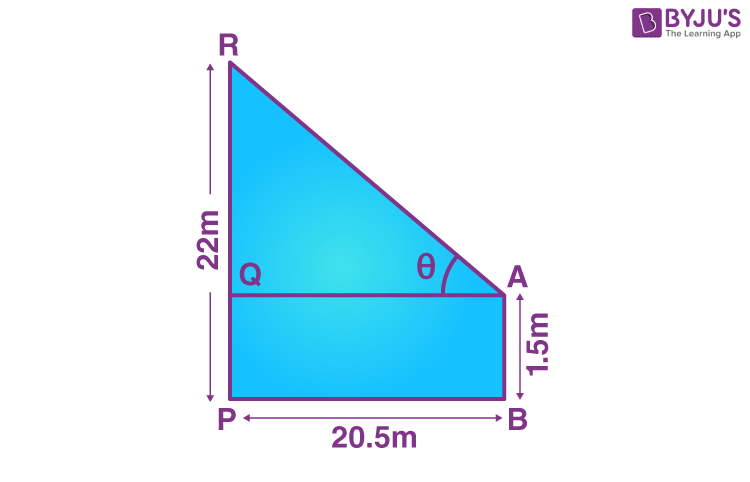From the above figure,

AB = PQ = 1.5 m

PB = QA = 20 m

PR = 22 m

QR = PR – PQ = 22 – 1.5 = 20.5 m

In the right triangle AQR,

tan θ = QR/AQ

tan θ = 20.5/20.5 = 1

⇒ tan θ = tan 45°

⇒ θ = 45°

Hence, the angle of elevation is 45°.

Q. 5: The angle of elevation of the top of a tower from two points distant s and t from its foot are complementary. Prove that the height of the tower is √st.

Solution:

Let BC = s; PC = t

Let the height of the tower be AB = h.

∠ABC = θ and ∠APC = 90° – θ

(∵ the angle of elevation of the top of the tower from two points P and B are complementary)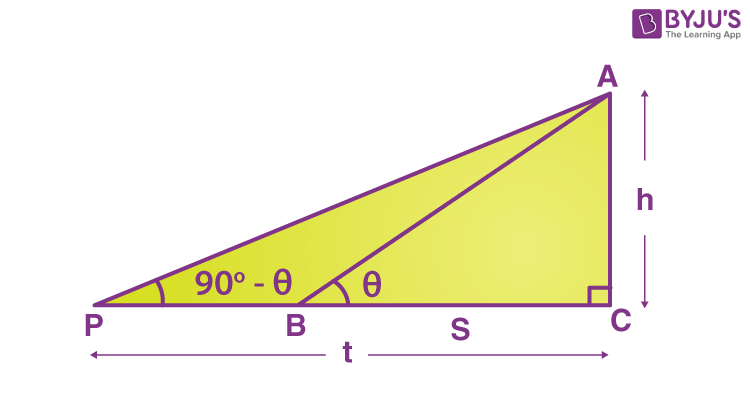In triangle ABC,

tan θ = AC/BC = h/s ………..(i)

In triangle APC,

tan (90° – θ) = AC/PC = h/t

cot θ = h/t ………..(ii)

Multiplying (i) and (ii),

tan θ × cot θ = (h/s) × (h/t)

1 = h2/st

h2 = st

h = √st

Hence, the height of the tower is √st.

Q.6: The angle of elevation of the top of a tower from a certain point is 30°. If the observer moves 20 metres towards the tower, the angle of elevation of the top increases by 15°. Find the height of the tower.

Solution:

Let AB be the height of the tower.

AB = h

The angle of elevation of the top of a tower from point P is 30°, i.e. ∠APB = 30°.

Given that, when the observer moves 20 metres towards the tower, the angle of elevation of the top increases by 15°.

Thus, PQ = 20 m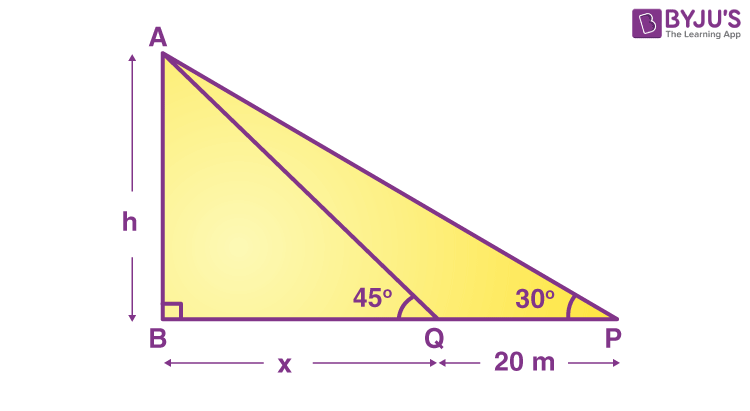Also, ∠AQB = 30° + 15° = 45°.

QB = x

In right triangle ABQ,

tan 45° = AB/QB

1 = h/x

h = x….(i)

In right triangle ABP,

tan 30° = AB/PB

1/√3 = h/(x + 20)

x + 20 = √3h  {from (i)}

h + 20 = √3h

√3h – h = 20

h = 20/(√3 – 1)

h = [20/(√3 – 1)] × [(√3 + 1)/(√3 + 1)]

= 20(√3 + 1)/(3 – 1)

= 20(√3 + 1)/2

= 10(√3 + 1)

Therefore, the height of the tower is 10(√3 + 1) m.

Q.7: A circus artist is climbing a 20 m long rope, which is tightly stretched and tied from the top of a vertical pole to the ground. Find the height of the pole, if the angle made by the rope with the ground level is 30°.

Solution:

Let AB be the vertical pole and AC be the length of the rope.

Also, the angle of elevation = ∠ACB = 30°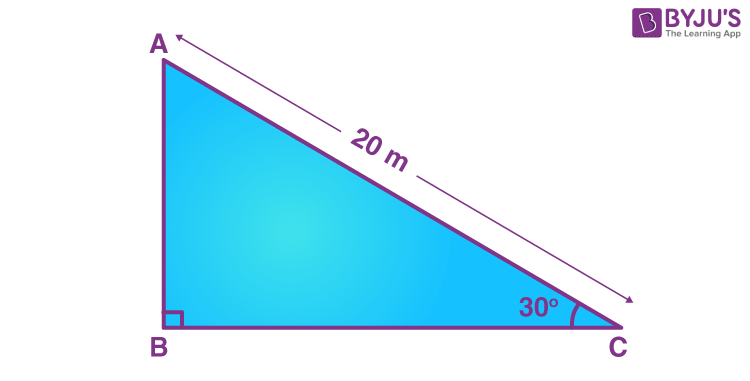In right triangle ABC,

sin 30 = AB/AC

1/2 = AB/20

AB = 20/2 = 10

Therefore, the height of the vertical pole is 10 m.

Q.8: From the top of a 7 m high building, the angle of elevation of the top of a cable tower is

60° and the angle of depression of its foot is 45°. Determine the height of the tower.

Solution:

Let AB be the height of the building and CE be the height of the tower.

Also, A be the point from where the elevation of the tower is 60° and the angle of depression of its foot is 45°.

EC = DE + CD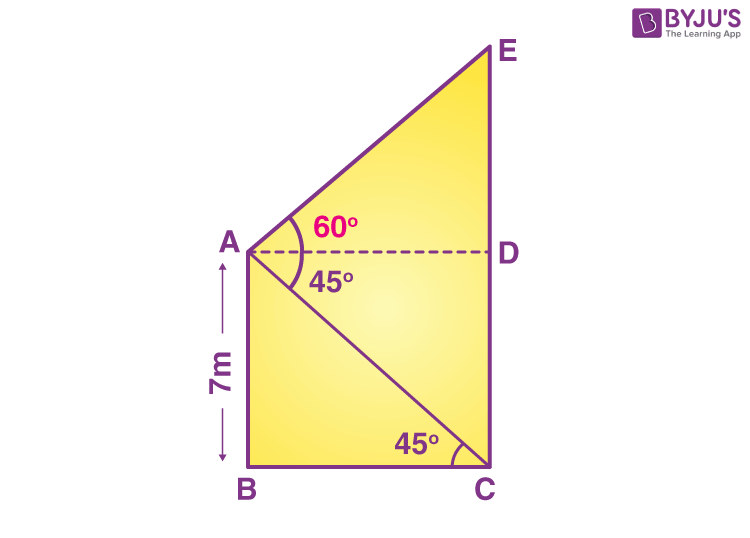From the figure,

CD = AB = 7 m

In right ΔABC,

tan 45° = AB/BC

1 = 7/BC

BC = 7 {since BC = AD}

√3 = DE/7

⇒ DE = 7√3 m

EC = DE + CD = (7√3 + 7) = 7(√3 + 1)

Therefore, the height of the tower is 7(√3 + 1) m.

## Video Lesson on Applications of Trigonometry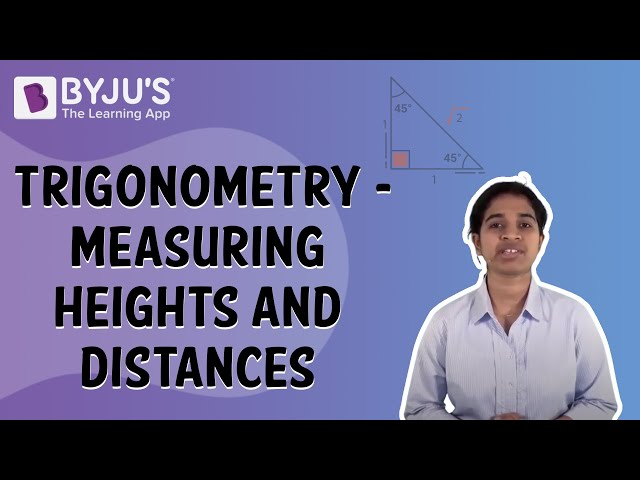### Practice Questions For Class 10 Maths Chapter 9 Some Applications of Trigonometry

1. The angle of elevation of the top of a hill at the foot of a tower is 60° and the angle of elevation of the top of the tower from the foot of the hill is 30°. If the tower is 50 m high, what is the height of the hill? [Answer: 150 m]
2. A bridge across the river makes an angle of 45° with the river bank. If the length of the bridge across the river is 150 m, what is the width of the river? [Answer: 75√2 m]
3. There is a small island in the middle of a 100 m wide river and a tall tree stands on the island. P and Q are the points directly opposite to each other on the two banks, and in a line with the tree. If the angles of elevation of the top of the tree from P and Q are 30° and 45° respectively, find the height of the tree. [Answer: 36.6.m]
4. From a point 20m away from the foot of a tower, the angle of elevation of the top of the tower is 30°. Find the height of the tower. [Answer: 11.56 m]
5. A flagstaff stands at the top of a 5m high tower. From a point on the ground, the angle of elevation of the top of the flagstaff is 60° and from the same point, the angle of elevation of the top of the tower is 45°. Find the height of the flagstaff. [Answer: 3.65 m]
6. A tower subtends an angle α at a point A in the place of its base and the angle of depression of the foot of the tower at a point b ft. just above A is β. Prove that the height of the tower is b tan α cot β.
7. A vertical tower stands on a horizontal plane and is surmounted by a flagstaff of height 7m. From a point on the plane, the angle of elevation of the bottom of the flagstaff is 30° and that of the top of the flagstaff is 45°. Find the height of the tower. [Answer: 9.55m]
8. The angle of elevation of a cloud from a point h metres above the surface of a lake is θ and the angle of depression of its reflection in the lake is φ. Prove that the height of the cloud above the lake is h[(tan φ + tan θ)/ (tan φ – tan θ)].
9. The angles of depression of the top and the bottom of an 8 m tall building from the top of a multi-storeyed building are 30° and 45°, respectively. Find the height of the multi-storeyed building and the distance between the two buildings. [Answer: 4(3 + √3) m]
10. A straight highway leads to the foot of a tower. A man standing at the top of the tower observes a car at an angle of depression of 30°, which is approaching the foot of the tower at a uniform speed. Six seconds later, the angle of depression of the car is found to be 60°. Find the time taken by the car to reach the foot of the tower from this point. [Answer: 3 sec]